Next:Entropy of a ContinuousUp:Entropy Rate and Entropy

## Entropy Series

This subsection deals with a measure of entropy commonly referred as entropy series, where in the probability distribution we have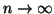. This quantity is given by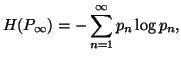where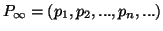with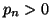and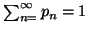.

Let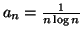and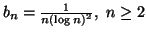be two sequences. Then it can easily be checked that the series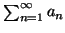diverges and the series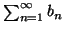converges. Let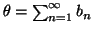be the sum of the series. Consider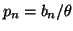, then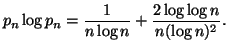In view of the fact that the seriesdiverges, we get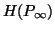as infinite. In order that the entropy series converges, we need some restrictions. If there is a convergent series of positive terms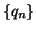such that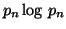also converges. Then by use of the inequality (1.9), we get the following bound: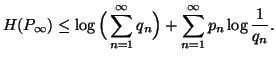The following properties give better restrictions to bound the entropy series.

Property 1.64. If for some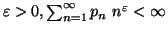, then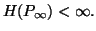Property 1.65. For each nondecreasing probability sequence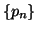i.e.,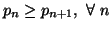, the entropy seriesconverges iff the series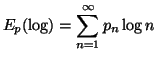converges.

Property 1.66. For each nondecreasing sequence, the following bound on the entropy series holds: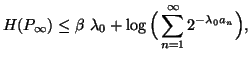where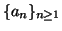is a nonnegative, nondecreasing sequence of numbers with the property that for some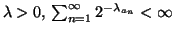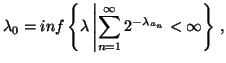and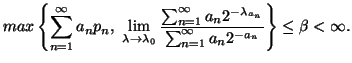Property 1.67. For each nondecreasing probability sequence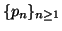, the following bound on the entropy series hold: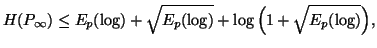where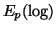is as given in property 1.65.

Property 1.68. (Maximum-Entropy Principle). The entropy seriesunder the constraints

(a)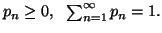(b)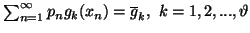is maximized when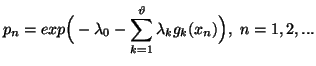and the maximum value is given by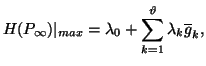where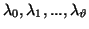are normalizing constants.

Note 1.6. Using the condition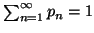, we get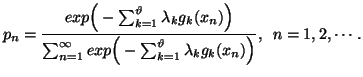(1.12)

By applying the conditions, we can calculate the constants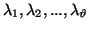. The probability distribution (1.12) is famous in the literature as Gibbs distribution''.

Particular cases: The property 1.69 admits the following interesting particular cases.

(i) The probability distribution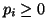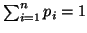that maximizes the corresponding entropy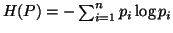is the uniform distribution given by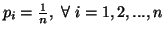.
(ii) The probability distribution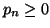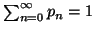that maximizes the corresponding entropy series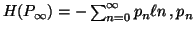subject to the constraint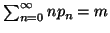is the geometric distribution given by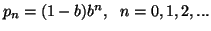, where b can be obtained by using the given constraints.
(iii) The probability distribution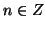(integers)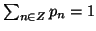that maximizes the corresponding entropy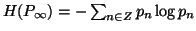subject to the constraints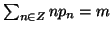and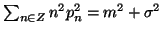is the discrete normal distribution given by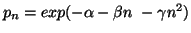, where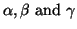can be obtained by using the given constraints.
Property 1.69. Let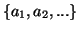be a sequence of real numbers with the property that for some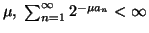, then the following bound on the entropy series hold: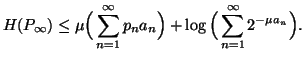Property 1.70. The following bound on the entropy series holds: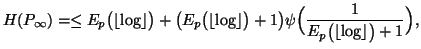where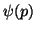is as given in (1.8).

The following properties are also worth emphasizing, whose details can be seen in Capocelli et al. (1988a;b) .

Property 1.71. The following bound on the entropy series hold: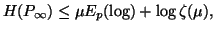where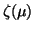is the Riemann zeta function and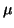is the unique solution of the equation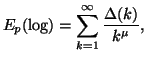and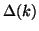is defined as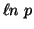when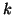is a power of a prime p; 0 otherwise.

Property 1.72. The following bound on the entropy series hold: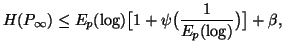where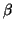is a bounded function of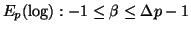, with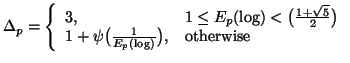Property 1.73. For each nonincreasing probability distribution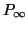and for each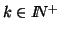, the following bound on the entropy series holds: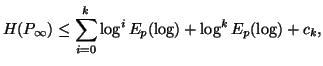where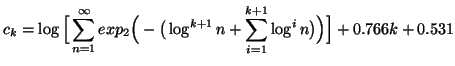is a constant independent of the probability distributionand for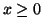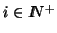, we have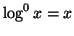and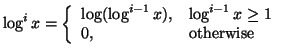Property 1.74. For each nonincreasing probability distribution, the following bound on the entropy series holds: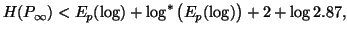where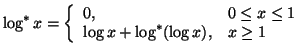Property 1.75. For each nonincreasing probability distributionand for each, the following limits hold:

(i)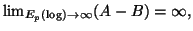(ii)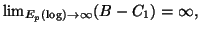(iii)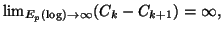(iv)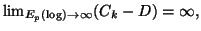where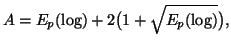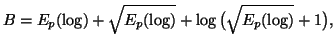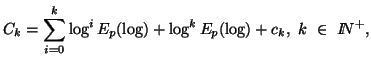and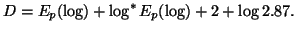Note 1.7. The good references for the sections 1.4, 1.5, 1.6 and 1.7 are the book by Csiszár and Körner (1981) , Guiasu (1977) , McEliece (1977) , etc..
21-06-2001
Inder Jeet Taneja
Departamento de Matemática - UFSC
88.040-900 Florianópolis, SC - Brazil